Categories

A MathsJam Puzzle

This week was MathsJam week, and I really liked the puzzle that @KathrynHTaylor shared with us. I’ve reproduced it below: Can you do it and have you got an answer?

• There are 50 seats on an aeroplane, and 50 people with numbered (in ascending order) tickets queing to board the plane. The first passenger doesn’t bother checking their seat number and sits randomly in a seat. The subsequent passengers sit in their allocated seated if it is free. If it has been taken, they then choose somewhere to sit (from the remaining free seats) at random. This process continues until all pasengers are seated. What is the probability that passenger 50 can sit in seat 50?
Categories

Summer Further Mathematics Taster Questions

Over the summer holidays I like my prospective Year 12 Further Mathematicians to look at some maths over the holiday (I do emphasise the importance of having a proper holiday too!). For the last couple of years I have given them these 3 questions (which I have found from various places over the years) to look at: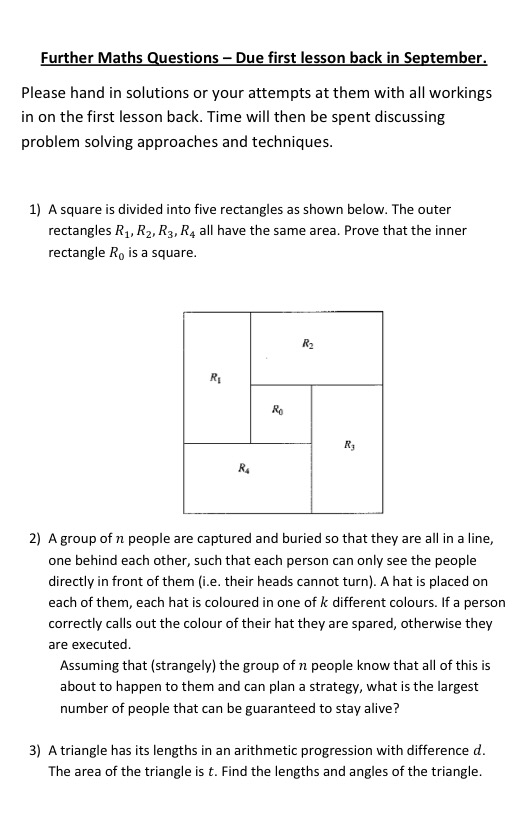I admit that these questions are not reflective of further mathematics questions and I say this to the students. In addition, I emphasise that I am not expecting full solutions, that they are hard questions and that they shouldn’t be worried if they get stuck. I explain that I am interested in seeing how they think mathematically and them building up the resilience to spend longer struggling through a problem and trying multiple approaches. For many of them this will be the first time they have come up against a problem that requires a bit more thought than “just do it”.

If you want, you can download the questions here – let me know what you think.

Categories

Barnsley’s Fern

Barnsley’s fern is a mathematically  generated image that resembles the Black Spleenwort fern as shown below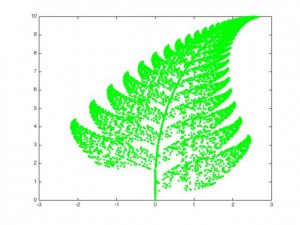This fractal was first described by Michael Barnsley in his book Fractals Everywhere in 1993. It is surprisingly easy to generate and is an example of an iterated function system. It is based on randomly applying one of four affine transformations.

\begin{align} f_1(x,y) &= \begin{pmatrix} 0.00 & 0.00 \\ 0.00 & 0.16 \end{pmatrix} \begin{pmatrix} x \\ y \end{pmatrix} + \begin{pmatrix} 0.00 \\ 0.00 \end{pmatrix} \\ f_2(x,y) &= \begin{pmatrix} 0.85 & 0.04 \\ -0.04 & 0.85 \end{pmatrix} \begin{pmatrix} x \\ y \end{pmatrix} + \begin{pmatrix} 0.00 \\ 1.60 \end{pmatrix} \\ f_3(x,y) &= \begin{pmatrix} 0.20 & -0.26 \\ 0.23 & 0.22 \end{pmatrix} \begin{pmatrix} x \\ y \end{pmatrix} + \begin{pmatrix} 0.00 \\ 1.60 \end{pmatrix} \\ f_4(x,y) &= \begin{pmatrix} -0.15 & 0.28 \\ 0.26 & 0.24 \end{pmatrix} \begin{pmatrix} x \\ y \end{pmatrix} + \begin{pmatrix} 0.00 \\ 0.44 \end{pmatrix} \end{align}

The initial point is set to be the origin, and subsequent points are generated by applying one of the above four transformations. Which transformation is chosen probabalistically – the probabilities are $$0.01,0.85,0.07$$ and $$0.07$$ for $$f_1,f_2,f_3$$ and $$f_4$$ respectively.

I wrote two (basic) functions in Matlab, one to generate the points and then one to plot them.

A video (generated using Matlab) showing the growth of the fern is below:

Here is the Matlab code I used to generate the video – it takes a relatively long time to run as it plots each frame separately.
Categories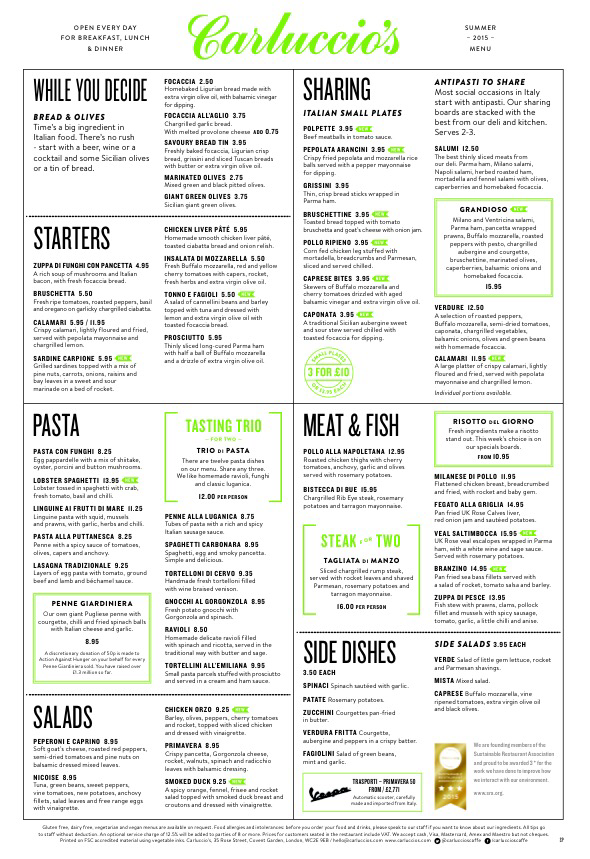For example on the above menu (from Carluccio’s, which is one of my favourite Italian restaurants) you could work out the most expensive and the cheapest three course combination. You could also compare it to the set menu and work out the most you could save – it’s always good to maximise savings! There’s also a multitude of the “I’ve bought 4 main courses, 3 desserts and 2 starters and it costs this much, what did I buy type?” problems. In a similar vein I have used menu prices when creating simultaneous equations that are harder than the typical GCSE integer valued questions. By creating some margin percentages for each meal, and some numbers representing the number of covers of each sold in a night a matrix multiplication problem can be created to find the profit made by a restaurant from one service. This could also lead on to discussions about the conditions necessary for the matrix multiplication ABc to be the same as the matrix multiplication BAc (where c is a cold vector containing the meal prices)…
I’d recently been led to think about menu pricing when a picture was posted on Twitter. It reminded me of a study that I had read a couple of years ago on the effect of price formatting on the sales in a restaurant. This study “Effects of Menu-price Formats on Restaurant Checks” by Yang, S, Kimes, S and Sessarego was published in the Cornell Hospitality Report in 2009. In this study diners were given menus with three different price formats and the effect of these formats on the total bills paid. As you would expect the party size had the most impact, dining duration and guests willingness to pay also had a large effect (the p value was less than 0.0001 for these three variables). The overall regression analysis combined the three different price formats into one variable, which didn’t seem to affect the total price paid significantly. However, the authors then performed a more detailed analysis of the three different price formats using a linear contrast analysis and they found that parties with a numeral only format (i.e. no $sign) spent$3.70 more than the average party and those who had been given the menus with the prices written in text spent \$1.85 less. This increase translated to a 8.15 percent increase in spend per person.  Perhaps this explains why more menus such as the Carluccio’s one above don’t include a £ sign when they list prices.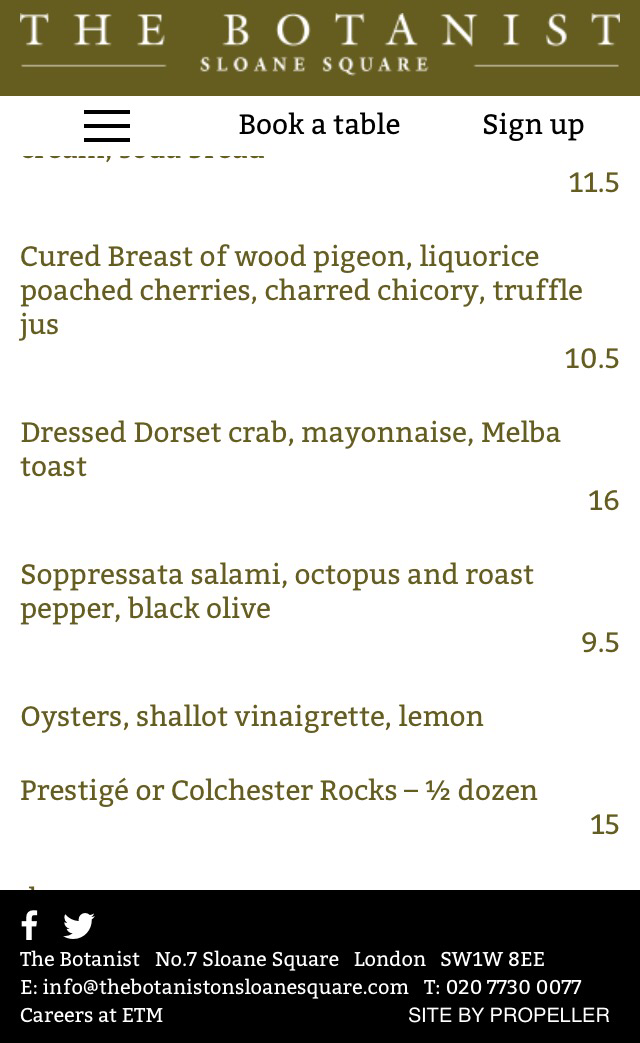I’m not sure how I feel about the use of 9.5 instead of 9.50… Though any discomfort I have with it is, I guess, only because we are used to having prices with two decimal points. Realistically there is no reason to have the trailing zeros. What do you think?